Search by Topic

Resources tagged with Cartesian equations of lines similar to Surprising Transformations:

Filter by: Content type:
Age range:
Challenge level:

There are 10 results

Broad Topics > Coordinates and Coordinate Geometry > Cartesian equations of lines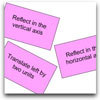Surprising Transformations

Age 14 to 16 Challenge Level:

I took the graph y=4x+7 and performed four transformations. Can you find the order in which I could have carried out the transformations?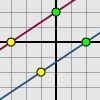Translating Lines

Age 11 to 14 Challenge Level:

Investigate what happens to the equation of different lines when you translate them. Try to predict what will happen. Explain your findings.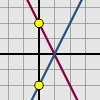Reflecting Lines

Age 11 to 14 Challenge Level:

Investigate what happens to the equations of different lines when you reflect them in one of the axes. Try to predict what will happen. Explain your findings.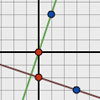Perpendicular Lines

Age 14 to 16 Challenge Level:

Position the lines so that they are perpendicular to each other. What can you say about the equations of perpendicular lines?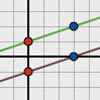Parallel Lines

Age 11 to 14 Challenge Level:

How does the position of the line affect the equation of the line? What can you say about the equations of parallel lines?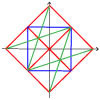Coordinate Designs

Age 11 to 14 Challenge Level:

This task requires learners to explain and help others, asking and answering questions.Enclosing Squares

Age 11 to 14 Challenge Level:

Can you find sets of sloping lines that enclose a square?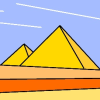Painting Between the Lines

Age 11 to 16 Challenge Level:

In abstract and computer generated art, a real object can be represented by a simplified set of lines. Can you create a picture using mathematical instructions?Close to Triangular

Age 14 to 16 Challenge Level:

Drawing a triangle is not always as easy as you might think!Kite in a Square

Age 14 to 16 Challenge Level:

Can you make sense of the three methods to work out the area of the kite in the square?